2018-05-15 18:23:49 zssyu0416 阅读数 8993
• ###### MATLAB图像处理

全面系统的学习MATLAB在图像处理中的应用

19892 人正在学习 去看看 魏伟

J = imadjust（I，[LOW_IN; HIGH_IN]，[LOW_OUT; HIGH_OUT]）将强度图像I中的值映射到J中的新值，使得LOW_IN和HIGH_IN之间的值映射到LOW_OUT和HIGH_OUT之间的值。低于LOW_IN和高于HIGH_IN的值被剪切; 也就是说，低于LOW_IN的值映射到LOW_OUT，高于HIGH_IN的值映射到HIGH_OUT。也可以使用空矩阵（[]）作为[LOW_IN; HIGH_IN]或[LOW_OUT; HIGH_OUT]指定[0 1]的默认值。如果省略参数，[LOW_OUT; HIGH_OUT]默认为[0 1]。

figure, imshow(I)
figure, imshow(J)
figure, imshow(K)
RGB2 = imadjust(RGB1,[.2 .3 0; .6 .7 1],[]);
figure, imshow(RGB1),

figure, imshow(RGB2)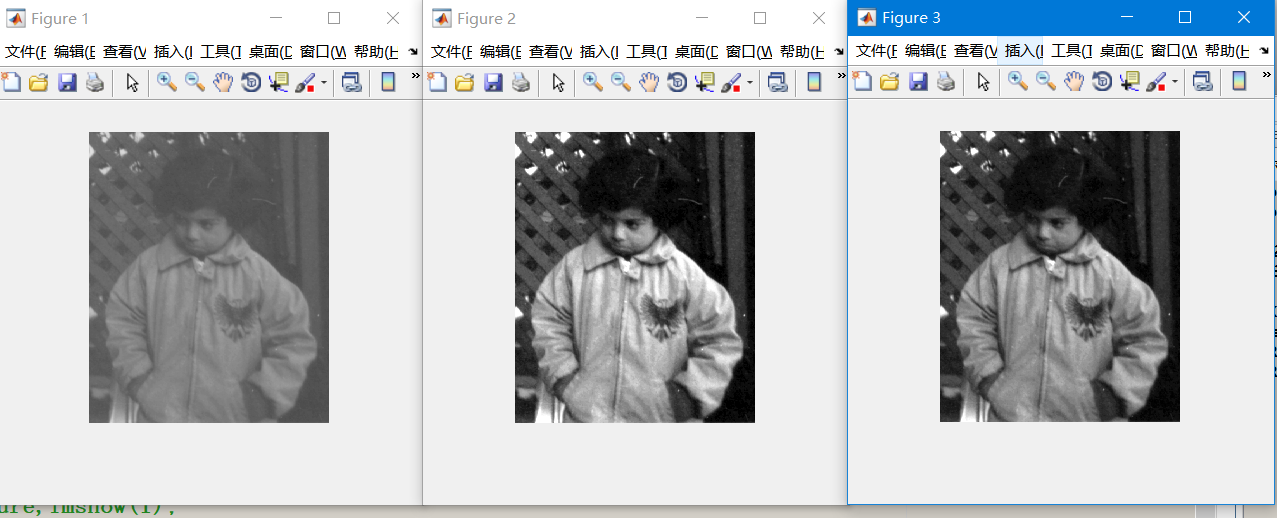2016-12-08 10:17:37 weicao1990 阅读数 1592
• ###### MATLAB图像处理

全面系统的学习MATLAB在图像处理中的应用

19892 人正在学习 去看看 魏伟

图像变换的操作一般可以分为下面两种：

1.基于邻域操作进行区域变换，比如卷积操作、特征提取、图像梯度计算、角点检测、图像模糊与蜕化等。

2.基于点操作进行像素的变换，比如图像亮度于对比度的调整。

下面我们对图像的亮度与对比度的调节进行讨论。

亮度与对比度的调节主要基于下面的公式，其中g(i,j)为处理前的图像，f(i,j)为处理后的图像：

g(i,j) = alpha * f(i,j) + beta,其中alpha>0,beta是增益变量。

这个公式是一个线性的变换，通过调整alpha和beta的值就会得到不同的效果。基本原理是这样的，图像f(i,j)乘以一个大于1的alpha值的时候，图像f(i,j)中的像素值之间的差异就会变大，因此对比度就会增强，如果alpha值在0~1之间对比度就会减小。后面的beta是一个增益变量，如果beta取正数的话，图像亮度就会变大，取负数的话图像亮度就会减小。（基本原理就是一次线性函数y=kx+b,调整k和b，y值就会随之变化）

下面是基于opencv的示例代码：

#include <opencv2/opencv.hpp>
#include <iostream>

using namespace cv;
using namespace std;

int main()
{
Mat src, dst;

if(!src.data)
{
cout << "could not load image ..." << endl;
}

char input_win[] = "input image";
namedWindow(input_win, CV_WINDOW_AUTOSIZE);
imshow(input_win, src);

//contrast and brightness changes
int height = src.rows;
int width = src.cols;

dst = Mat::zeros(src.size(), src.type());

float alpha = 1.2;
float beta  = 30;

for(int row = 0; row < height; row++)
{
for(int col = 0; col < width; col++)
{
if(src.channels() == 3)
{
float b = src.at<Vec3b>(row, col); //blue
float g = src.at<Vec3b>(row, col); //green
float r = src.at<Vec3b>(row, col); //red

dst.at<Vec3b>(row, col) = saturate_cast<uchar>(b*alpha + beta);
dst.at<Vec3b>(row, col) = saturate_cast<uchar>(g*alpha + beta);
dst.at<Vec3b>(row, col) = saturate_cast<uchar>(r*alpha + beta);
}
else if(src.channels() == 1)
{
float v = src.at<uchar>(row, col);
dst.at<uchar>(row, col) = saturate_cast<uchar>(v*beta + beta);
}
}
}

char output_title[] = "contrast and brightness changes demo";
namedWindow(output_title, CV_WINDOW_AUTOSIZE);
imshow(output_title, dst);

waitKey(0);

return 0;
}

上面的代码主要用到opencv函数下面对此进行说明：

Mat new_image = Mat::zero(image.size(), image.type);//创建一张跟原图大小和类型一致的空白图像、像素初始为0。
saturate_cast<uchar>(value);//确保值大小为0~255。
Mat.at<Vec3b>(y,x)[index] = value; //给每个像素点每个通道赋值。

2014-12-15 10:01:11 xyz128 阅读数 592
• ###### MATLAB图像处理

全面系统的学习MATLAB在图像处理中的应用

19892 人正在学习 去看看 魏伟

# 图像对比度增强

1、图像增强处理并不能增加原始图像的信息，只能增强对某种信息的辨别能力，而这种处理肯定会损失一些其它信息；

2、强调根据具体应用而言，更“好”更“有用”的视觉效果图像；

3、图像增强处理最大的困难——增强后图像质量的好坏主要依靠人的主观视觉来评定，也就是说，难以定量描述。

## 图像对比度的增强技术可以分为两类：全局处理和局部处理

### 局部对比度增强的技术有：

（1）基于统计的局部增强方法
（2）同态滤波方法
（3）多尺度形态学方法

2019-05-10 15:15:20 qq_40090859 阅读数 358
• ###### MATLAB图像处理

全面系统的学习MATLAB在图像处理中的应用

19892 人正在学习 去看看 魏伟

# 例子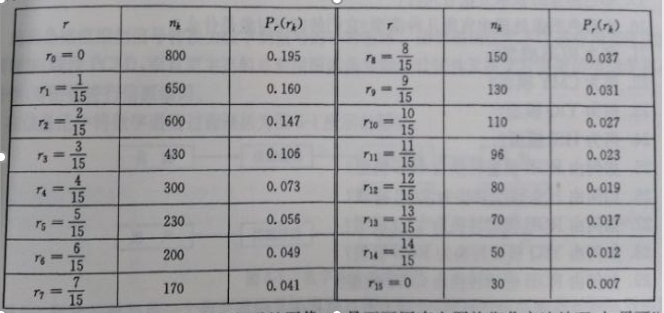# 解答

## 处理直方图的步骤

### 步骤

(1).直方图输入

``````clc
count=[800,650,600,430,300,230,200,170,150,130,110,96,80,70,50,30];
N=4096;
x=16;
figure(1);
hold on;
bar(count)
OUT1=zeros(1,x);
``````

(2)计算概率

``````PDF=count/N;%PDF±íÊ¾Ã¿¸ö»Ò¶È¼¶±ð³öÏÖµÄ¸ÅÂÊ£¬Ò»¹²ÓÐ256ÐÐ
``````

(3)计算累加概率:

``````CDF=cumsum(PDF);%CDF±íÊ¾ÖðÐÐÏà¼ÓµÄ¸ÅÂÊ£¬Ò²¾ÍÊÇÀÛ¼Ó¸ÅÂÊ
``````

(4)计算均衡后的灰度级:

``````for i=1:x
for j=1:count(i)
%d=round(CDF(i)*x-1);
OUT1(round(CDF(i)*x-1))=OUT1(round(CDF(i)*x-1))+1;
end
end
``````

(5).输出灰度级直方图

``````figure(2);
hold on;
bar(OUT1
``````

## 实验结果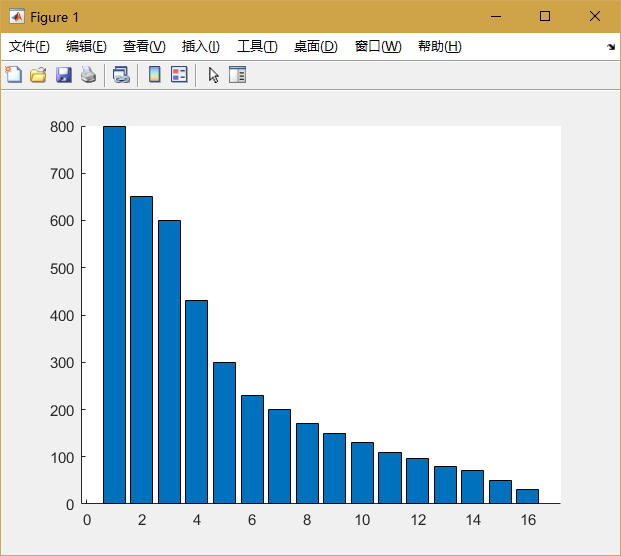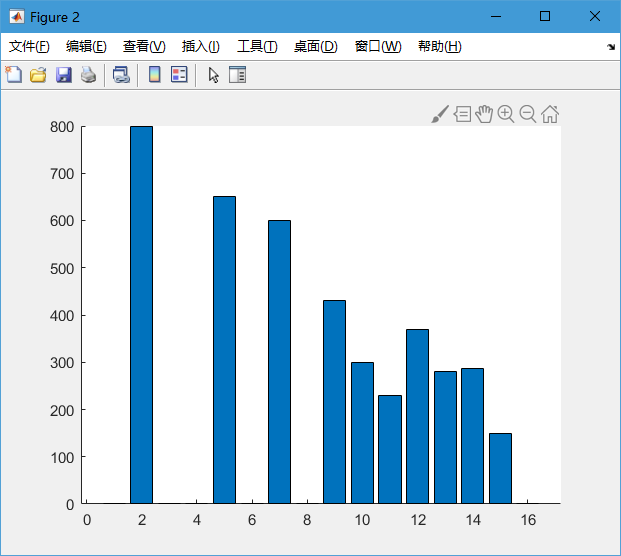# 图像的直方图均衡化

## 代码

``````clear all;
clc;
close all;

[m,n,d]=size(f);%灰度图1维，彩色图3维

if d==1
f1=f;%复制后新的图片f1，作为改变后的图片
elseif d==3
f=rgb2gray(f);
f1=f;
end

figure
imhist(f)

[count,x]=imhist(f);%count表示每个灰度级别有多少个像素，x表示有多少个灰度级别

PDF=count/(m*n);%PDF表示每个灰度级别出现的概率，一共有256行

CDF=cumsum(PDF);%CDF表示逐行相加的概率，也就是累加概率

for i=1:256
xiangsuxushu=find(f==i);%原本灰度级别为i的像素在第几位
changdu=length(xiangsuxushu);
for j=1:changdu
f1(xiangsuxushu(j))=round(CDF(i)*256-1);%每一个原本灰度级别为i的像素，
%灰度级别改为累加出现概率*256
%再取整
end
end

figure
imhist(f1)
figure
subplot(1,2,1);
imshow(f)
subplot(1,2,2);
imshow(f1)
``````

## 结果

1. 原始直方图：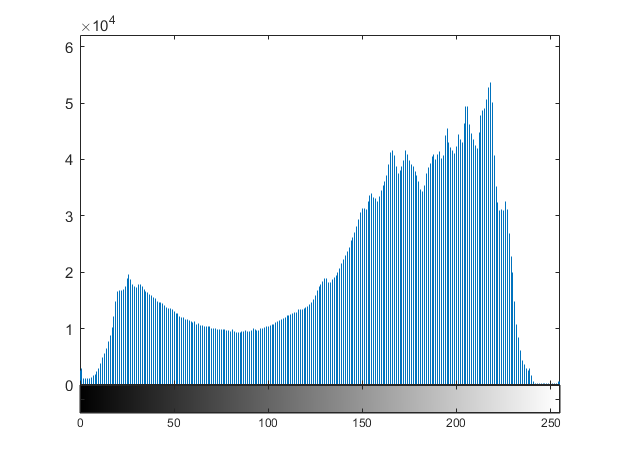2. 均衡化后的直方图：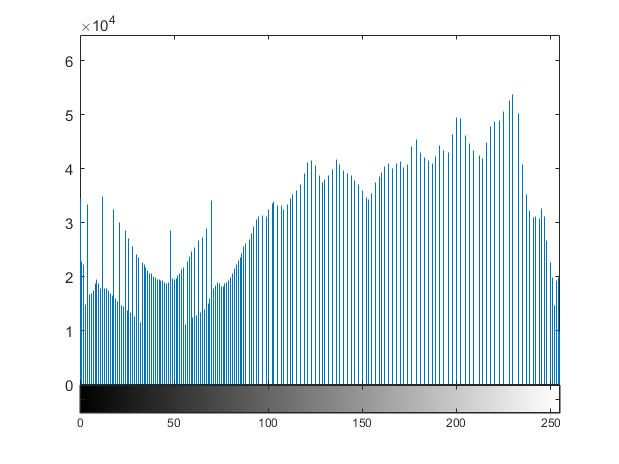3. 图片对比：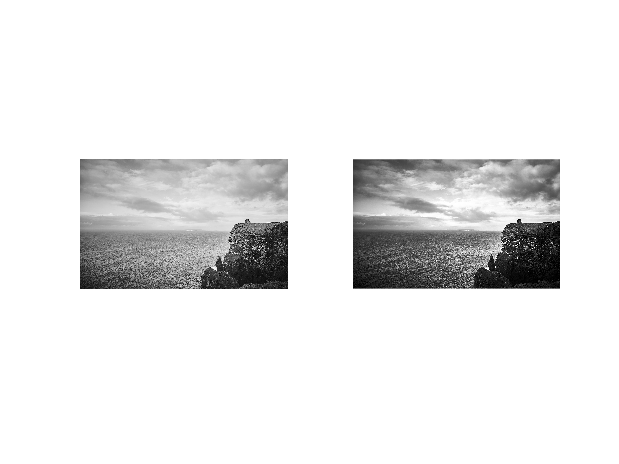可见，直方图均衡化使灰度被拉开。

# MATLAB自带的直方图处理工具

## 代码

``````close all;
clc;
%函数histeq（）进行直方图均衡化处理

J=histeq(I);  %直方图均衡化

figure,
subplot(121),imshow(uint8(I));
title('原图')

subplot(122),imshow(uint8(J));
title('均衡化后')
figure,

subplot(121),imhist(I,64);
title('原图像直方图');

subplot(122),imhist(J,64);
title('均衡化后的直方图');

``````

## 结果

1. 直方图对比：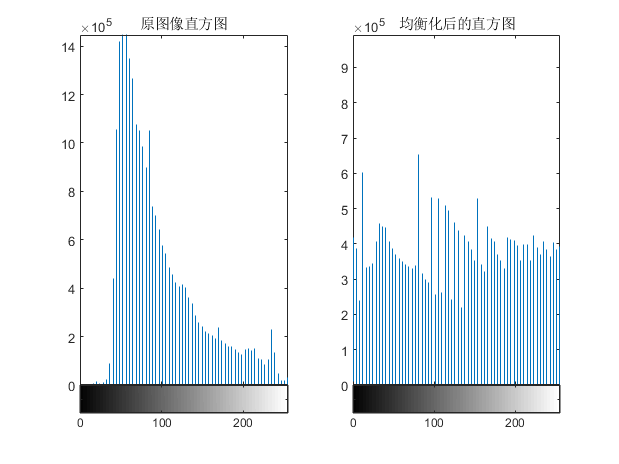2. 图片对比：# 总结

2019-07-23 21:41:18 weixin_41059269 阅读数 333
• ###### MATLAB图像处理

全面系统的学习MATLAB在图像处理中的应用

19892 人正在学习 去看看 魏伟

直方图均衡化是图像处理领域中利用图像直方图对对比度进行调整的方法，其主要思想是将一副图像的直方图分布变成近似均匀分布，从而增强图像的对比度。这种方法通常用来增加许多图像的全局对比度，尤其是当图像的有用数据的对比度相当接近的时候。通过这种方法，亮度可以更好地在直方图上分布，这样就可以用于增强局部的对比度而不影响整体的对比度。
这种方法对于背景和前景都太亮或者太暗的图像非常有用，这种方法尤其是可以带来X光图像中更好的骨骼结构显示以及曝光过度或者曝光不足照片中更好的细节。
这种方法的一个主要优势是它是一个相当直观的技术并且是可逆操作，如果已知均衡化函数，那么就可以恢复原始的直方图，并且计算量也不大。这种方法的一个缺点是它对处理的数据不加选择，它可能会增加背景噪声的对比度并且降低有用信号的对比度。
直方图均衡化和规定化都是通过全局直方图调节来改变图像的对比度，其实就是根据一个表来做映射。均衡化一句话，就是拿图像本身的累积分布表(cdf)来做映射。规定化就是图像B拿图像A的累积分布表(cdf)来做映射。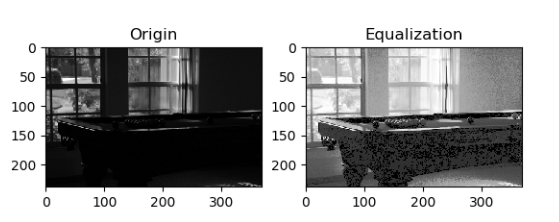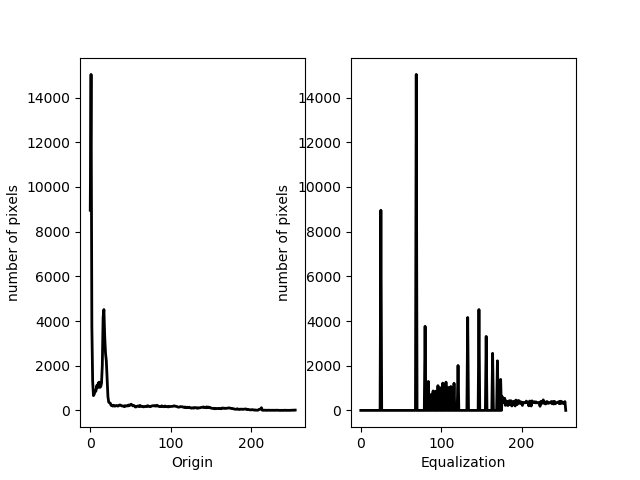Python代码：

``````#coding:utf-8
#*********************************************************************************************************
'''

1)以灰度图的方式加载图片;
2)求出原图的灰度直方图，计算每个灰度的像素个数在整个图像中所占的百分比;
3)计算图像各灰度级的累积概率密度分布；
4)求出新图像的灰度值。
'''

import cv2
import numpy as np
import matplotlib.pyplot as plt

def Origin_histogram( img ):
#建立原始图像各灰度级的灰度值与像素个数对应表
histogram = {}
for i in range( img.shape ):
for j in range( img.shape ):
k = img[i][j]
if k in histogram:
histogram[k] += 1
else:
histogram[k] = 1

sorted_histogram = {}#建立排好序的映射表
sorted_list = sorted( histogram )#根据灰度值进行从低至高的排序

for j in range( len( sorted_list ) ):
sorted_histogram[ sorted_list[j] ] = histogram[ sorted_list[j] ]

return sorted_histogram

def equalization_histogram( histogram, img ):

pr = {}#建立概率分布映射表

for i in histogram.keys():
pr[i] = histogram[i] / ( img.shape * img.shape )

tmp = 0
for m in pr.keys():
tmp += pr[m]
pr[m] =  max( histogram ) * tmp

new_img = np.zeros( shape = ( img.shape, img.shape ), dtype = np.uint8 )

for k in range( img.shape ):
for l in range( img.shape ):
new_img[k][l] = pr[img[k][l]]

return new_img

def GrayHist( img ):
# 计算灰度直方图
height, width = img.shape[:2]
grayHist = np.zeros(, np.uint64)
for i in range(height):
for j in range(width):
grayHist[img[i][j]] += 1
return grayHist

if __name__ == '__main__':
#读取原始图像
#计算原图灰度直方图
origin_histogram = Origin_histogram( img )
#直方图均衡化
new_img = equalization_histogram( origin_histogram, img )

origin_grayHist = GrayHist(img)
equaliza_grayHist = GrayHist( new_img )
x = np.arange(256)
# 绘制灰度直方图
plt.figure( num = 1 )
plt.subplot( 2, 2, 1 )
plt.plot(x, origin_grayHist, 'r', linewidth=2, c='black')
plt.title("Origin")
plt.ylabel("number of pixels")
plt.subplot( 2, 2, 2 )
plt.plot(x, equaliza_grayHist, 'r', linewidth=2, c='black')
plt.title("Equalization")
plt.ylabel("number of pixels")
plt.subplot( 2, 2, 3 )
plt.imshow( img, cmap = plt.cm.gray )
plt.title( 'Origin' )
plt.subplot( 2, 2, 4 )
plt.imshow( new_img, cmap = plt.cm.gray )
plt.title( 'Equalization' )
plt.show()

``````